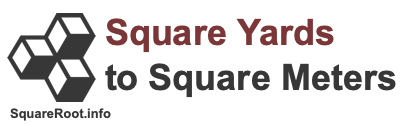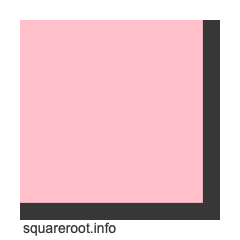50 square yards to square metersHere we will explain and show you how to convert 50 square yards to square meters. Before we continue, note that 50 square yards to square meters is also known as 50 sq yards to sq meters, 50 sqyd to sqm, 50 yd2 to m2, and 50 yd² to m².

To create a formula to calculate 50 square yards to square meters, we start with the fact that one yard equals 0.9144 meters. Therefore, this formula is true:

yards × 0.9144 = meters

However, we are dealing with square yards and square meters, which means yards and meters to the 2nd power. Thus, we take both sides of the formula above to the 2nd power to get this result:

yards² × 0.9144² = meters²
yards² × 0.83612736 = meters²
yd² × 0.83612736 = m²

Now we know that one square yard is equal to 0.83612736 square meters, and that the formula to convert square yards to square meters is as follows:

yd² × 0.83612736 = m²

Below is an illustration showing you how one square meter fits into one square yard. The cut out in pink is how one square meter fits into the entire square yard. One square meter is about 83.61 percent of a square yard.When we enter 50 square yards into our newly created formula, we get the answer to 50 square yards converted to square meters:

yd² × 0.83612736 = m²
50 × 0.83612736
= 41.806368
50 yd² ≈ 41.81 m²

Square Yards to Square Meters Converter
Please enter another square yards area in the box below to have it converted to square meters.

Convert  square yards to square meters.

51 square yards to square meters
Here is the next area in square yards on our list that we have converted to square meters for you.